Next: Application to Gd Up: ANGULAR-MOMENTUM PROJECTION OF CRANKED Previous: Introduction

# Angular-Momentum Projection

A deformed solution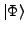of Eq. (1) is a superposition of eigenstates of the angular-momentum operator. The angular-momentum conserving wave function can be obtained from the stateby applying the angular-momentum projector: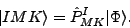(6)

The AMP operator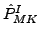is a projector onto angular momentum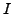with projection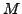along the laboratory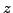axis, and reads:[14,1]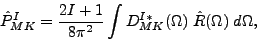(7)

where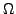represents the set of three Euler angles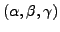,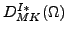are the Wigner functions, and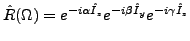is the rotation operator.

The state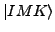is no longer a product wave function, but a complicated superposition of Slater determinants. The operatoris not a projector in the mathematical sense. It extracts from the intrinsic wave function the component with a projection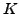along the intrinsicaxis of the nucleus. Sinceis not a good quantum number, all these components must be mixed, and the mixing coefficients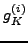must be determined by the minimization of energy. This-mixing is taken into account by assuming the following form for the eigenstates: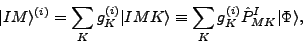(8)

and by solving the following Hill-Wheeler (HW) equation: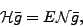(9)

where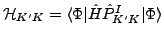and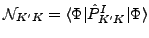denote the Hamiltonian and overlap kernels, respectively.Next: Application to Gd Up: ANGULAR-MOMENTUM PROJECTION OF CRANKED Previous: Introduction
Jacek Dobaczewski 2006-10-30